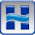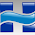## Wednesday, June 3, 2009

Written by Chris Goodell, P.E., D. WRE | WEST Consultants

Why do you sometimes see the water surface elevation and the total energy increase in the downstream direction? The energy grade line should always slope downhill. Water surface doesn’t necessarily have to though (i.e. hydraulic jumps, transition from narrow cross section to wide cross section). In this case, I’m not sure what the problem is without seeing the model firsthand, but the EGL indicates localized errors. This usually occurs from either not enough cross sections, or HTAB computation points that are too coarse, or the computation points don’t go low enough in the cross section. Try maxing out the number of HTAB points for each cross section, and decrease the increment as well. Also, use the invert of the channel as the starting computation point (there’s a button to do that automatically). Run the model. If there are still some problems, try interpolating some cross sections in the areas where there is increasing energy. Also, turning on the critical depth variable in your profile plot can help to explain what’s going on.

1.I'm a bit of a newcomer to HEC-RAS, and found your blog. I know this is not quite related to this post, however here goes:

Can you back out the EGL slope from HEC-RAS outputs of the EGL itself? I can't seem to find a way to relate them. I.e.: Se(i,t)~=(EGL(i+1,t)-EGL(i,t))/L, or any other combinations thereof. Thanks!

2.Hi Michael-

Yes, you can. If you go to the Profile Summary Table, you can get just about any variable you can think of, including Energy Grade Line Elevation (E.G. Elev), Reach Length (Length Chnl, Left, Rght, Wtd.), etc. While in the table, click Options...Define Table, and just pick the variables you want. The Energy Slope at cross sections is also provided (E.G. Slope), however this is a discrete calculation of Energy slope, in that RAS computes this for a cross section by backing out of Manning's Equation (as opposed to a reach length averaged approximation like you show).

3.Thanks so much, that last comment cleared things up for me. I may pester you again, in the future. :) Would these sort of questions fit better here, or on the http://forum.rasmodel.com/ site?

4.The forum gets more looks, and potantially more responses. On the blog, you'll most likely only get a response from me. The downside is that depending on my schedule or workload it may take a while to follow up with a response on the blog.

5.Hey Chris,

How is the E.G. Slope different from the Friction Slope? I'm assuming that the E.G. Slope calculation also includes head losses due to contraction and expansion losses.

Thanks,
Katy

1.Also, how does Hecras account for energy losses through hydraulic jumps?

2.Generally speaking, they are one in the same. However, in RAS terminology, the Friction Slope and the E.G. slope are slightly different. You are correct that the E.G. slope represents the total energy loss from one cross section to the next and includes the friction losses plus losses from contraction and expansion, while the friction slope is strictly from losses due to roughness. When looking at output, however, the friction slope represents an average between 2 cross sections and is exactly the friction slope that is used to compute friction losses. The E.G. Slope is a discrete slope at one cross section and is back-calculated from the computed water surface elevation and discharge using Manning's equation.

3.With regard to hydraulic jumps, RAS abandons the energy equation at hydraulic jumps and uses the momentum equation instead. Friction losses are captured through a Friction Force term, and form losses (contraction/expansion) are captured by change in momentum. Chapter 2 of the Hydraulic Reference Manual can provide more detail on this.

6.Thank you! I really appreciate your help.
I have one more question, also related to the energy grade elevation. If I calculate the energy grade line slope by hand, I find it deviates from the headloss (as reported in the profile output table) significantly in an area where there is an abrupt drop in the channel invert. Why would this be? Shouldn't headloss always equal the slope of the energy grade line (as calculated from the EGL elevation reported in the profile output table)? Thanks again, Katy

7.Does it go through critical depth? If so, perhaps RAS is using the momentum equation there. Otherwise, I'm not sure, without doing some testing, but I'll leave that up to you :). Let me know what you discover!

8.Yeah, I'm running it in mixed flow, and there is a hydraulic jump in the cross section with the seemingly spurious EGL elevation. How would a hydraulic jump affect the EGL calculation? Wouldn't the energy losses be accounted for in the friction slope and thus overall headloss?
Thanks again, your help has been very useful (and heartening).

9.Yes...you would think so. Form losses are also accounted for in the momentum equation.

10.I dont think it is always correct that the HEC-RAS "E.G. slope represents the total energy loss from one cross section to the next and includes the friction losses plus losses from contraction and expansion". You can examine the "C&E Loss" column from HEC-RAS, and reduce it to a gradient by dividing by the section spacing (lets call this the C&E Slope). You can do the same from the "Total Loss" column (lets call this the "Total Loss Slope"). I am finding that "Total Loss Slope n/e to "E.G Slope", when there are C&E Losses. The E.G slope is equal to the "Total loss slope" minus "C&E Slope" (ie losses due to friction only). This makes sense as manual says E.G calculated by rearranging Mannings Equation. This is confusing, but it means that the E.G. slope is not the total loss slope, and also that the stream power (which should be called "Unit stream power dissipation") reported by HEC-RAS is only the power dissipated due to friction (not C&E losses). Similarly the shear stress reported by HEC-RAS is the shear stress due to friction losses.

11.I have the problem "The energy equation could not be balanced within the specified number of iterations.
During the standard step iterations, when the assumed water surface was set equal to critical depth, the calculated water surface came back below critical depth. This indicates that there is no valid subcritical answer. The program defaulted to critical depth. ", Where I have a mixed flow I have already interpolated sections and the problem follows the error I get in the section where there is the change from subcritical to supercritic, which is what I could do to correct

12.Hi! How an HECRAS rating curve counts for energy level on a 2D boundary conditions? If the water velocity is important on the structure, modeled by a rating curve...is HECRAS using the water level or the energy level (upstream from the structure) to calculate dicharge over the structure? Which cells are used to do this? Is there a way to change the location to read the water level to be use in the discharge calculation using the rating curve?
Thx!

13.how can I find out the energy slope

1.You can find it in the profile output table.